# Square Root: Sign, Rules & Problems

An error occurred trying to load this video.

Try refreshing the page, or contact customer support.

Coming up next: The Square Root Property

### You're on a roll. Keep up the good work!

Replay
Your next lesson will play in 10 seconds
• 0:05 Basic Characteristics…
• 1:03 Perfect Squares
• 1:28 Recognizing Perfect Squares
• 2:46 Simplifying Square…
• 6:25 Adding and Subtracting…
• 9:01 Lesson Summary

Want to watch this again later?

Timeline
Autoplay
Autoplay
Speed

#### Recommended Lessons and Courses for You

Lesson Transcript
Instructor: David Liano
In this lesson, you will learn how to recognize square root notation. You will also learn properties of square roots and discover how to simplify expressions that include square roots.

## Basic Characteristics of Square Roots

Mathematics is the language of science, and like any language, there are terms and rules that you must master to speak it fluently. We'll explore the terms and rules that you'll need to follow any basic conversation involving square root signs. Like any sign, square root signs are trying to tell us something. They're telling us to find the square roots of whatever numbers are beneath them, and the numbers beneath a square root sign is called a radicand.As for square roots, a number r is a square root of a number x if r^2 = x. Based on this definition, a positive number actually has two square roots: a positive number and the negative of that positive number. For example, the numbers 5 and -5 are square roots of 25 because 5^2 = 25 and (-5)^2 = 25. You can think of every positive root as having a negative, evil twin.

However, we're going to focus on the positive and limit our conversation to principal square roots, the positive square roots of positive numbers. For example, the principal square root of 25 is 5. Also, we won't cover the square roots of negative numbers, which would require a discussion of imaginary numbers, a conversation for another day. Now, let's learn to speak the language of square roots.

## Recognizing Perfect Squares

When speaking any language, it's good to know the simpler version of what you want to say. Perfect squares provide us with a way to find the simpler versions of square root expressions. A radicand is a perfect square if its principal square root is an integer. For example, 16 is a perfect square because its principal square root is 4. The number 26 is not a perfect square because its principal square root (about 5.1) is not an integer.

To visualize the idea of perfect squares, look at the following squares. You can display any perfect square (r^2) as an r-by-r grid, where r is an integer. For example, 4 equal-sized square units can be displayed as a square in a 2-by-2 grid. A 3-by-3 grid gives you 9 squares, a 4-by-4 square grid gives you 16 squares, and so on. However, you can't display a number that's not a perfect square, like 26, in this same fashion. There's no r-by-r square grid that'll give you 26 squares.Recognizing perfect squares is important because it helps us simplify more complex square root expressions. Instead of saying, 'The square root of 16,' it's simpler to say '4.'

## Simplifying Square Root Expressions

A square root expression is considered simplified once it meets two conditions:

1) The radicands have no perfect square factors other than 1

and

2) There are no square root signs in the denominator

What our first condition really means is we're looking for perfect squares under the square root sign. We can pull those perfect squares out from under the square root sign and write them as integers.

For example, to simplify the square root of 200, you'd want to find the perfect square factors of 200. We can write 200 as 2*100. Is 2 a perfect square? No, its principal square root is not an integer. Is 100 a perfect square? Yes, its principal square root is 10! Let's pull that 100 out from under the radical sign and write it as 10. So, the square root of 200 can be simplified to 10 times the square root of 2.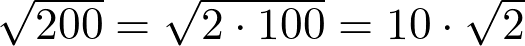That one didn't seem too difficult, but what if we were asked to simplify the square root of 2 times the square root of the quantity 200 divided by 3. Don't be intimidated. In addition to recognizing perfect squares, there are two properties that'll help us accomplish this task.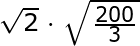If a is greater than 0 and b is greater than 0, then we can use the following properties to simplify square root expressions: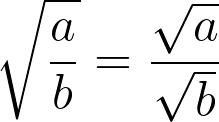First, we have the quotient property, where the square root of a / b equals the square root of a divided by the square root of b.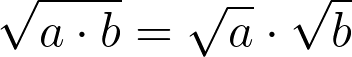We also have the product property, where the square root of a*b is the same as the square root of a times the square root of b.

We can use the quotient property to help with the square root of the quantity 200 divided by 3 part of our problem. Following this property, we can rewrite this part as the square root of 200 divided by the square root of 3. We know from earlier that the square root of 200 can be simplified to 10 times the square root of 2. So let's put that in our expression, too.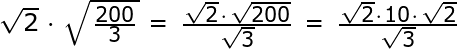Now, we have the square root of 2 times 10 times the square root of 2 in the numerator and the square root of 3 in the denominator.

To simplify the numerator, we can use the product property to write the square root of 2 times the square root of 2 as the square root of the quantity 2 times 2. This gives us the square root of 4, so we now have 10 times the square root of 4 in the numerator. Don't forget that 4 is a perfect square, so we can rewrite the square root of 4 as 2. This gives us 20 in our numerator.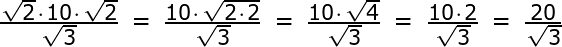Are we done? No, remember that there can't be a square root sign in the denominator, so how do we get rid of that square root of 3?

We have to rationalize the denominator. This is a fancy way of saying that we need to multiply by some version of 1 to get rid of square root signs in the denominator. In this case, we multiply our entire expression by the square root of 3 divided by the square root of 3.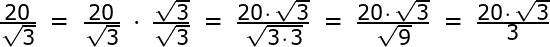Multiplying our denominator by the square root of 3 gives us the square root of 3 times 3 (remember the product property). This gives us the square root of 9 in our denominator, which is just 3.

Our final, simplified version is 20 times the square root of 3 all over 3. The radicands have no perfect square factor other than 1 and there are no square root signs in the denominator, so we're done!

## Adding and Subtracting Square Roots

Are you feeling confident? Even if you don't know the solution yet, the answer should always be yes, so we're going to introduce one more concept: adding and subtracting square roots.

To unlock this lesson you must be a Study.com Member.

### Register to view this lesson

Are you a student or a teacher?

### Unlock Your Education

#### See for yourself why 30 million people use Study.com

##### Become a Study.com member and start learning now.
Back
What teachers are saying about Study.com

### Earning College Credit

Did you know… We have over 160 college courses that prepare you to earn credit by exam that is accepted by over 1,500 colleges and universities. You can test out of the first two years of college and save thousands off your degree. Anyone can earn credit-by-exam regardless of age or education level.